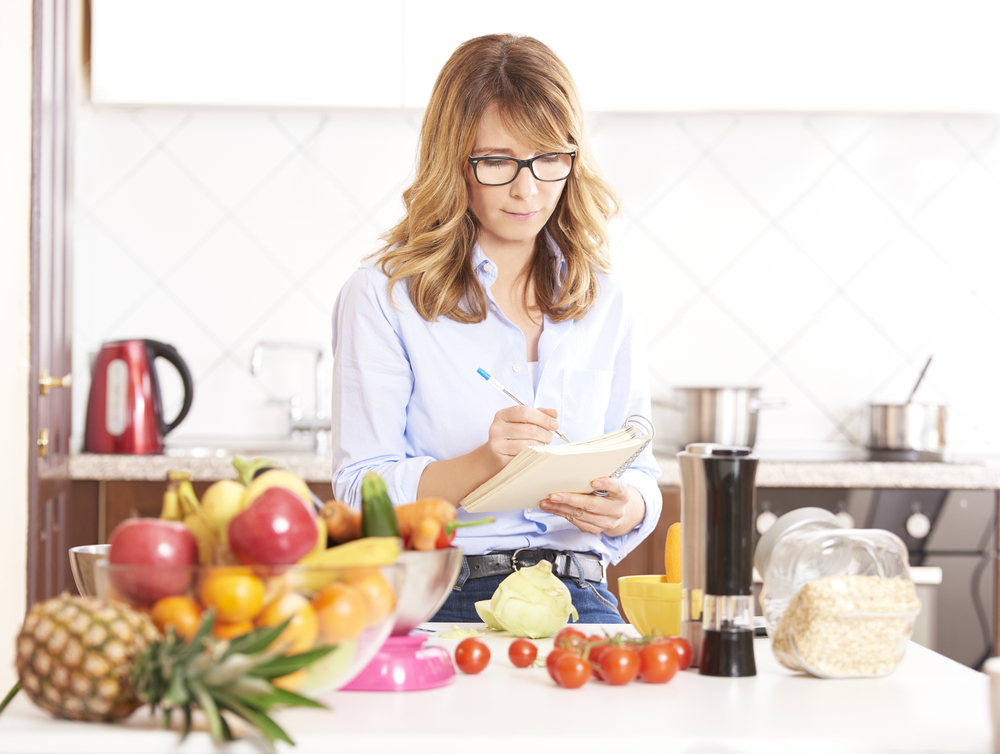# Calculating carb content with food reference guidesReference lists or books such as Carbs & Cals are an essential tool for working out the carbohydrate content of the food you eat. These are useful when there are no food labels to work from or when you are eating out.

With Carbs & Cals books, you can use them both as a quick visual guide to give a rough estimate of your portion size, and as a source of carbohydrate values so that you can accurately work out how much carbohydrate is in the portion of food that you are eating.

### Visual guide

To get a close estimate of your portion size of a particular food, you would look up the food you are eating in the Carbs & Cals book or app and then visually compare it to the portion size you are having.

You would then use the carbohydrate value of that portion to work out your bolus insulin dose.

### Using reference lists for exact calculations

You can use reference lists to work out the exact amount of carbohydrate in the food you are eating. To do this you will need some digital scales to weigh out your portion.

### Worked example for calculating carb content using reference lists

Let’s look at how we would work out how much carbohydrate is in a 75 gram portion of boiled potatoes using the following carbohydrate reference values:

We will use the following calculation:

As we know the carbohydrate value and the weight for boiled potatoes from the reference guide, we can do the calculation:

A 75 gram portion of boiled potatoes will have 12 grams of carbohydrate.

The video below gives you a step-by-step guide for calculating the carbohydrate content of food using both a visual guide and then working out the exact measure

In the next topic we’ll look at using handy measures in the home for carb counting.